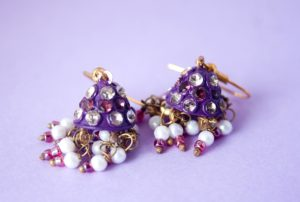# 2.1: Mark-Up Problems

$$\newcommand{\vecs}{\overset { \rightharpoonup} {\mathbf{#1}} }$$ $$\newcommand{\vecd}{\overset{-\!-\!\rightharpoonup}{\vphantom{a}\smash {#1}}}$$$$\newcommand{\id}{\mathrm{id}}$$ $$\newcommand{\Span}{\mathrm{span}}$$ $$\newcommand{\kernel}{\mathrm{null}\,}$$ $$\newcommand{\range}{\mathrm{range}\,}$$ $$\newcommand{\RealPart}{\mathrm{Re}}$$ $$\newcommand{\ImaginaryPart}{\mathrm{Im}}$$ $$\newcommand{\Argument}{\mathrm{Arg}}$$ $$\newcommand{\norm}{\| #1 \|}$$ $$\newcommand{\inner}{\langle #1, #2 \rangle}$$ $$\newcommand{\Span}{\mathrm{span}}$$ $$\newcommand{\id}{\mathrm{id}}$$ $$\newcommand{\Span}{\mathrm{span}}$$ $$\newcommand{\kernel}{\mathrm{null}\,}$$ $$\newcommand{\range}{\mathrm{range}\,}$$ $$\newcommand{\RealPart}{\mathrm{Re}}$$ $$\newcommand{\ImaginaryPart}{\mathrm{Im}}$$ $$\newcommand{\Argument}{\mathrm{Arg}}$$ $$\newcommand{\norm}{\| #1 \|}$$ $$\newcommand{\inner}{\langle #1, #2 \rangle}$$ $$\newcommand{\Span}{\mathrm{span}}$$$$\newcommand{\AA}{\unicode[.8,0]{x212B}}$$

### Learning OUTCOME

• Use mathematical notation to solve markup problemsBlue Boat Design Earrings

Ana runs Blue Boat Designs, where she makes colorful beaded earrings. She sells her earrings to boutiques around Los Angeles for$18$. A shop called Treasure Hunt purchases Ana’s earrings for$18$, marks them up$50\%$ and then sells them to shop customers for$27$.

Applications of mark-up are very common in retail settings. The price a retailer pays for an item is called the wholesale price. The retailer then adds a mark-up to the wholesale price to get the list price, the price he sells the item for. The mark-up is usually calculated as a percent of the wholesale price. The percent is called the mark-up rate. To determine the amount of mark-up, multiply the mark-up rate by the wholesale price. We summarize the mark-up model in the box below.

### Mark-up

The mark-up is the amount added to the wholesale price.

amount of mark-up = mark-up rate$\cdot$ wholesale price

list price = wholesale price + mark up

The list price should always be more than the wholesale price, or it would be a mark-down, not a mark-up.

The mark-up rate can be less than, equal to, or greater than$100\%$.

### example

Adam’s art gallery bought a photograph at the wholesale price of$\text{\250}$. Adam marked the price up$\text{40%}$. Find the amount of mark-up and the list price of the photograph.

Solution

 ⓐ Identify what you are asked to find. What is the amount of mark-up? Choose a variable to represent it. Let$m=$ the amount of each mark-up. Write a sentence that gives the information to find it. The mark-up is$40\text{%}$ of the wholesale price. Translate into an equation.Simplify.$m=100$ Check if this answer is reasonable. Yes. The markup rate is less than$50\text{%}$ and$\text{\100}$ is less than half of$\text{\250}$. Write a complete sentence that answers the question. The mark-up on the photograph was$\text{\100}$.
 ⓑ Identify what you are asked to find. What is the list price? Choose a variable to represent it. Let$p=$ the list price. Write a sentence that gives the information to find it. The list price is the wholesale price plus the mark-up. Translate into an equation.Simplify.$p=350$ Check if this answer is reasonable. Yes. The list price,$\text{\350}$, is more than the wholesale price,$\text{\250}$. Write a complete sentence that answers the question. The list price of the photograph was$\text{\350}$.

### try it

[ohm_question hide_question_numbers=1]146778[/ohm_question]

### TRY IT

[ohm_question hide_question_numbers=1]978[/ohm_question]

### EXAMPLE

A very common mark-up strategy in retail stores is to simply double the price they paid for the item. Let’s walk through how you would calculate the mark-up rate for this situation.

Braided Salon purchases natural hair dyes for$38$ from a beauty supply wholesaler. If the owner plans to double the price before selling it to customers, what is the mark-up rate?

Solution

If Braided Salon purchases the hair dye for$38$, they will charge customers$56$$(2 \cdot 38 = 56)$. The mark-up in this case is the difference between the final price tag and the wholesale cost:$56 - 38 = 38$.

amount of mark-up = mark-up rate$\cdot$ wholesale price$38 = x \cdot 38$$x = 1$$1 = 100%$

The mark-up rate is$100%$ when doubling the wholesale cost.

The next question refers to profit, which, in this case, is the same as mark-up amount.

### TRY IT

[ohm_question hide_question_numbers=1]154749[/ohm_question]

Mark-up is one type of percent of change problem. In the next video we show an example of how to calculate the percent increase of a salary, which is similar to the process of marking-up the price of an item.A YouTube element has been excluded from this version of the text. You can view it online here: http://pb.libretexts.org/afm-2/?p=106